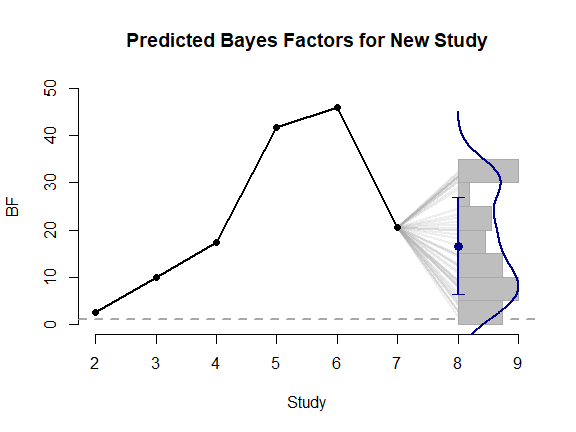# metaBMA: Bayesian Model Averaging for Random- and Fixed-Effects Meta-Analysis

## Overview

Fixed-effects meta-analyses assume that the effect size $$d$$ is identical in all studies. In contrast, random-effects meta-analyses assume that effects vary according to a normal distribution with mean $$d$$ and standard deviation $$\tau$$. Both models can be compared in a Bayesian framework by assuming specific prior distribution for $$d$$ and $$\tau$$. Given the posterior model probabilities, the evidence for or against an effect (i.e., whether $$d = 0$$) and the evidence for or against random effects can be evaluated (i.e., whether $$\tau = 0$$). By using Bayesian model averaging (i.e., inclusion Bayes factors), both types of tests can be performed by marginalizing over the other question. Most importantly, this allows to test whether an effect exists while accoungting for uncertainty whether study heterogeneity exists or not.

## Defining and Plotting Priors

To fit a meta-analysis model, prior distributins on the total effect $$d$$ and the heterogeneity $$\tau$$ are required. The package metaBMA leaves the user the freedom to choose from several predefined distributions or even define an owen prior density function. The function prior facilitates the construction and visual inspection of prior distributions to check whether they meet the prior knowledge about the field of interest.

# load package
library(metaBMA)
data(towels)

# Half-normal (truncated to > 0)
p1 <- prior("halfnorm", c(mean=0, sd=.3))
p1
## Prior density function (class='prior'): 'halfnorm' with mean=0, sd=0.3
p1(1:3)
##  1.028186e-02 5.940600e-10 5.129732e-22
plot(p1)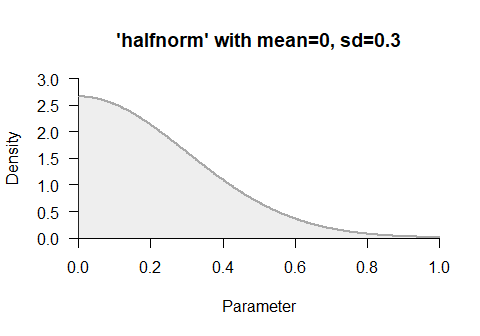# custom prior
p1 <- prior("custom",  function(x) x^3-2*x+3, "d", 0, 1)
plot(p1, -.5, 1.5)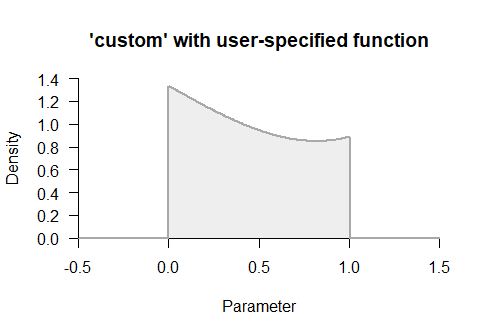# field- and effect-specific default priors
plot_default(field = "psychology", effect = "ttest")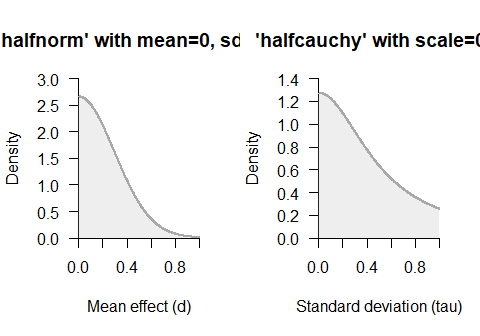## Bayesian Meta-Analysis

The functions meta_fixed() and meta_random() fit Bayesian meta-analysis models. The model-specific posteriors for $$d$$ can then be averaged by bma() and inclusion Bayes factors be computed by inclusion().

The fixed-effects meta-analysis assumes that the effect size is identical across studies. This model requires only one prior distribution for the overall effect $$d$$:

# Fixed-effects
mf <- meta_fixed(towels$logOR, towels$SE, towels$study, d = "halfnorm",d.par = c(0, .3)) mf ## ### Bayesian Fixed-Effects Meta-Analysis ### ## ## Prior on d: 'halfnorm' with mean=0, sd=0.3 ## Bayes factor (d ~ prior vs. d = 0) = 23.86745 ## ## ## # Posterior summary statistics: ## Mean Median SD q025 q975 HPD95lower ## d 0.2123145 0.2119299 0.07535458 0.06553894 0.3612395 0.06343137 ## HPD95upper ## d 0.3590283 # plot posterior distribution plot_posterior(mf)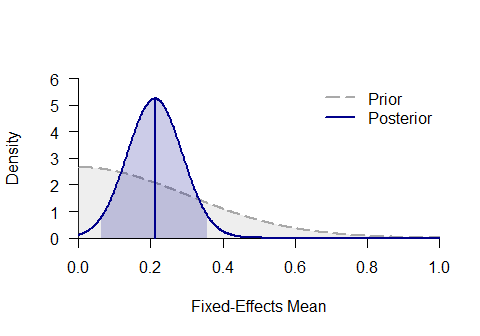In contrast, the random-effects meta-analysis assumes that the effect size varies across studies. Specifically, it is assumed that study effect sizes follow a normal distribution with mean $$d$$ and standard deviation $$\tau$$. This model requires two prior distributions for both parameters: # Random-effects mr <- meta_random(towels$logOR, towels$SE, towels$study,
tau = "halfcauchy",tau.par = .5,
d = "halfnorm",d.par = c(0,.3))
## Loading required namespace: rjags
mr
## ### Bayesian Random-Effects Meta-Analysis ###
##
##    Prior on d:      'halfnorm' with mean=0, sd=0.3
##    Prior on tau:    'halfcauchy' with scale=0.5
##    Bayes factor (d ~ prior vs. d = 0)      = 3.223879
##    Bayes factor (tau ~ prior vs. tau = 0)  = 0.2496883
##
##
## # Posterior summary statistics:
##          Mean    Median         SD       q025      q975  HPD95lower
## d   0.1939056 0.1926766 0.09064462 0.02569888 0.3764285 0.008268133
## tau 0.1393656 0.1079237 0.12453674 0.00491838 0.4583430 0.000000000
##     HPD95upper
## d    0.3523002
## tau  0.3818086
# plot posterior distribution
plot_posterior(mr, main = "Total effect size")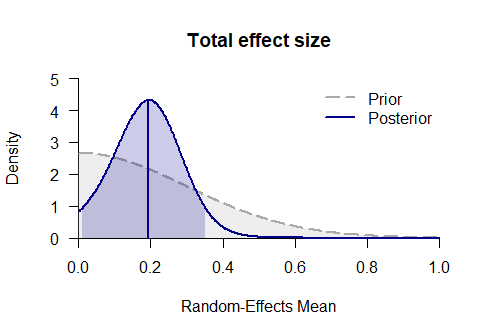plot_posterior(mr, "tau", main = "Heterogeneity tau")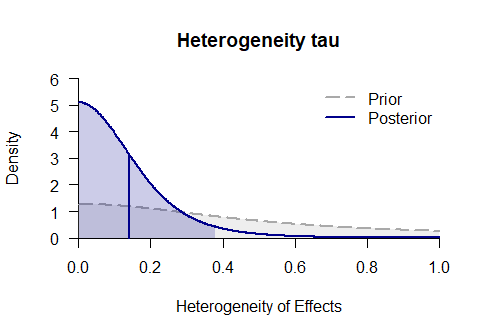## Model-Averaging for Meta-Analysis

The most general functions in metaBMA are meta_bma() and meta_default(), which fit random- and fixed-effects models, compute the inclusion Bayes factor for the presence of an effect and the averaged posterior distribution of the mean effect $$d$$ (which accounts for uncertainty regarding study heterogeneity).

mb <- meta_bma(towels$logOR, towels$SE, towels$study, d = "halfnorm", d.par = c(0, .3), tau = "halfcauchy", tau.par = .5) mb ## ### Bayesian Model Averaging of Meta-Analysis ### ## Mean effect d: ## Prior density function (class='prior'): 'halfnorm' with mean=0, sd=0.3 ## Prior density function (class='prior'): 'halfnorm' with mean=0, sd=0.3 ## ## # Bayes factors: ## d_10_fixed d_10_random d_10_averaged tau_10_random ## d_10 23.86745 3.223879 10.47099 0.2496883 ## ## # Model posterior probabilities: ## prior posterior marginal ## fixed.H0 0.25 0.03060407 0.003783434 ## fixed.H1 0.25 0.73044097 0.090300908 ## random.H0 0.25 0.05657240 0.006993774 ## random.H1 0.25 0.18238256 0.022547079 ## ## # Posterior summary statistics: ## Mean Median SD q025 q975 ## Averaged 0.2086364 0.2086511 0.07899123 0.05274583 0.3639548 ## Fixed Effects 0.2123145 0.2119299 0.07535458 0.06553894 0.3612395 ## Random Effects 0.1939056 0.1926766 0.09064462 0.02569888 0.3764285 ## HPD95lower HPD95upper ## Averaged 0.050199779 0.3612915 ## Fixed Effects 0.063431367 0.3590283 ## Random Effects 0.008268133 0.3523002 plot_posterior(mb, "d", -.1, 1.4)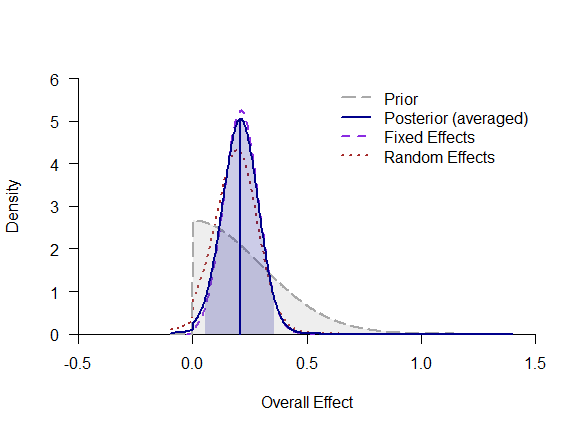plot_forest(mb)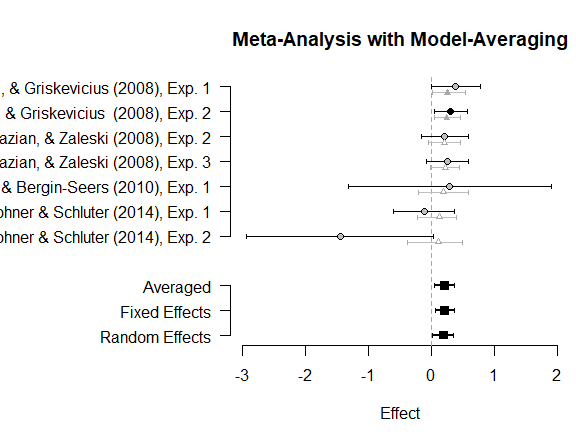Future versions of the package will have a function that uses sensible default priors. These priors are defined separately per field (psychology, medicince) and the type of effect size (t-test, log-odds ratio, correlations): md <- meta_default(towels$logOR, towels$SE, towels$study,
field = "psychology", effect = "logOR")
md

## Predicted Bayes Factor for New Study

Often, it is of interest to judge how much additional evidence future studies can contribute to the present knowledge. Conditional on the outcome of the model averaging for meta-analysis, the function predictive() samples new data sets from the posterior and performes a model selection for each replication. Thereby, a distribution of predicted Bayes factors is obtain that represents the expected evidence one expects when running a new study:

mp <- predictive(mb, SE = .2, resample = 50)
plot(mp)